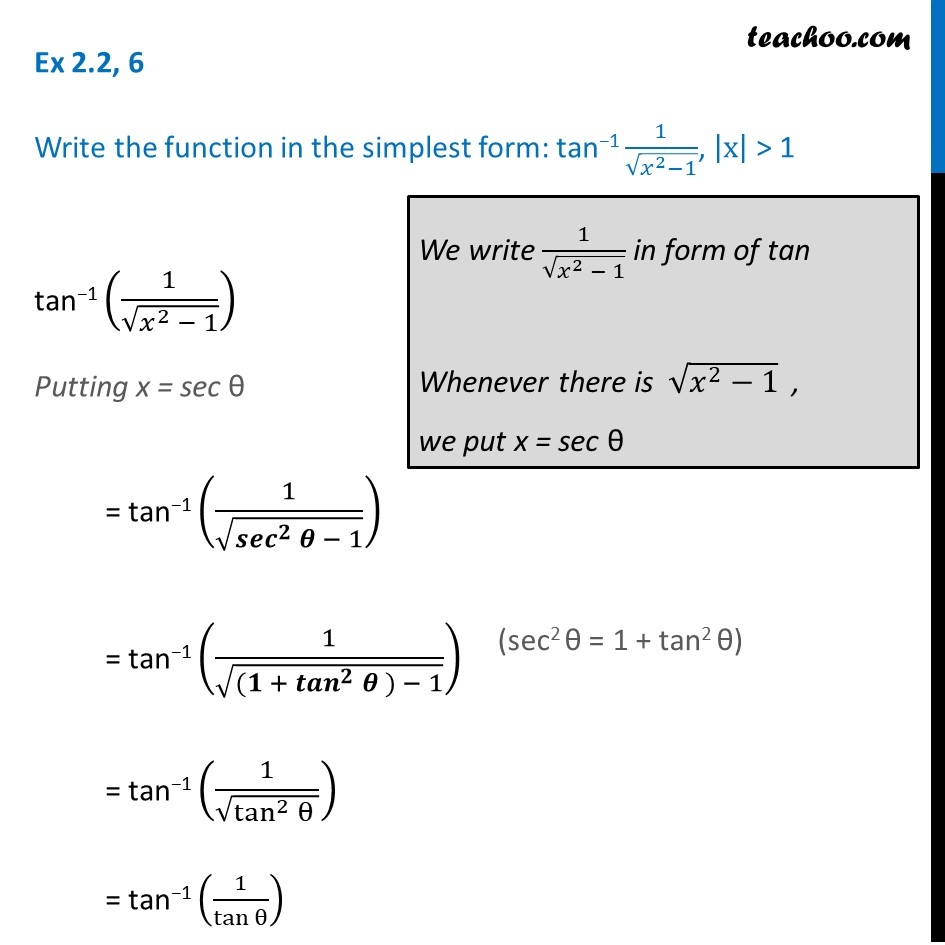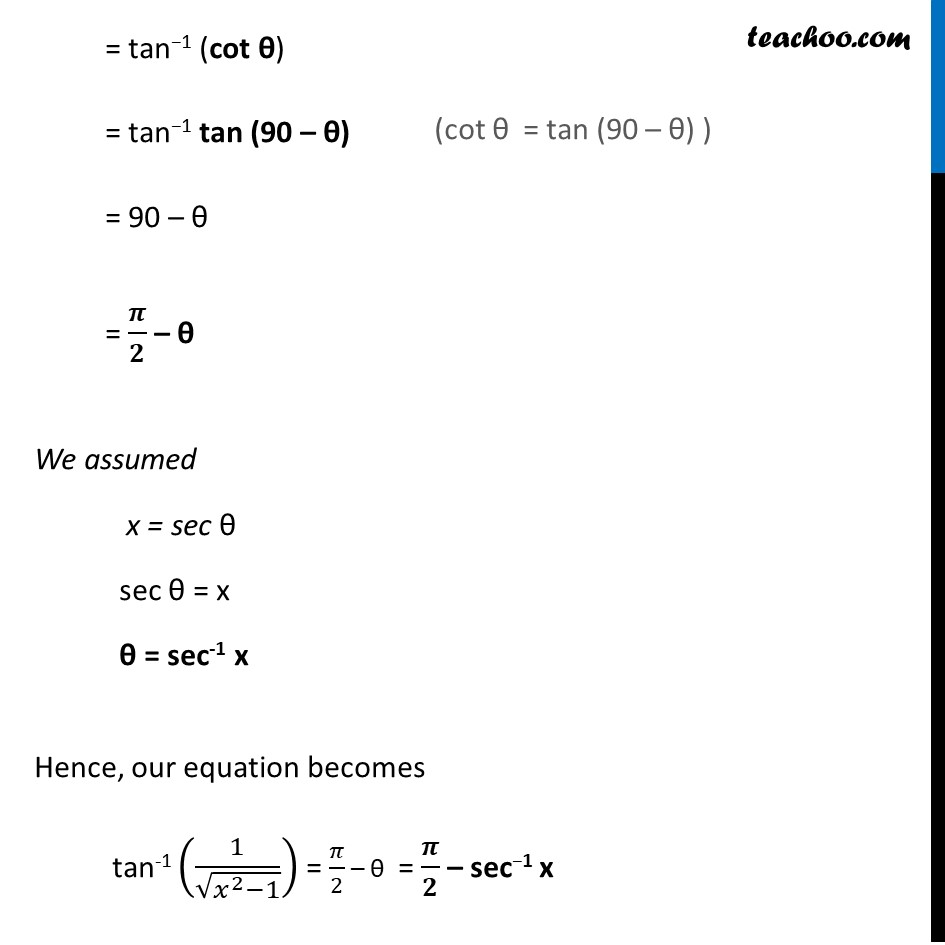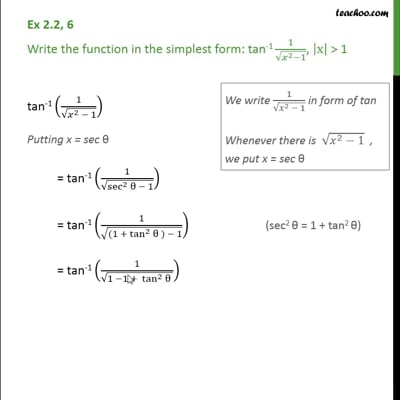Not clear how to approach

Chapter 2 Class 12 Inverse Trigonometric Functions
Concept wiseThis video is only available for Teachoo black users

Introducing your new favourite teacher - Teachoo Black, at only ₹83 per month

### Transcript

Ex 2.2, 6 Write the function in the simplest form: tan−1 1/√(𝑥^2−1), |x| > 1 tan−1 (1/√(𝑥^2 − 1)) Putting x = sec θ = tan−1 (1/√(〖𝒔𝒆𝒄〗^𝟐⁡𝜽 − 1)) = tan−1 (1/√(〖(𝟏 + 〖𝒕𝒂𝒏〗^𝟐〗⁡𝜽 ) − 1)) = tan−1 (1/√(tan^2⁡θ )) = tan−1 (1/tan⁡θ ) We write 1/√(𝑥^2 − 1) in form of tan Whenever there is √(𝑥^2−1) , we put x = sec θ = tan−1 (cot θ) = tan−1 tan (90 – θ) = 90 – θ = 𝝅/𝟐 – θ We assumed x = sec θ sec θ = x θ = sec-1 x Hence, our equation becomes tan-1 (1/√(𝑥^2−1)) = 𝜋/2 – θ = 𝝅/𝟐 – sec−1 x (cot θ = tan (90 – θ) )